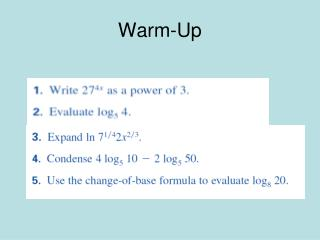DownloadDownload PresentationWarm-Up

# Warm-Up

Télécharger la présentation## Warm-Up

- - - - - - - - - - - - - - - - - - - - - - - - - - - E N D - - - - - - - - - - - - - - - - - - - - - - - - - - -
##### Presentation Transcript

1. Warm-Up

2. Exponential Equations • One way to solve exponential equations is to use the property that if 2 powers w/ the same base are equal, then their exponents are equal. • For b>0 & b≠1 if bx = by, then x=y

3. Solve by equating exponents • 43x = 8x+1 • (22)3x = (23)x+1 rewrite w/ same base • 26x = 23x+3 • 6x = 3x+3 • x = 1 Check → 43*1 = 81+1 64 = 64

4. Your turn! • 24x = 32x-1 • 24x = (25)x-1 • 4x = 5x-5 • 5 = x Be sure to check your answer!!!

5. When you can’t rewrite using the same base, you can solve by taking a log of both sides • 2x = 7 • log 2x = log 7 • x log 2 = log 7 • x = ≈ 2.807

6. 4x = 15 • log4x = log15 • x log 4 = log15 • x = log15/log4 • ≈ 1.953

7. 102x-3+4 = 21 • -4 -4 • 102x-3 = 17 • log 102x-3 = log 17 • (2x-3) log 10 = log 17 • 2x – 3 = log17/log 10 • 2x = (log 17/log10) +3 • ≈ 2.115

8. 5x+2 + 3 = 25 • 5x+2 = 22 • log 5x+2 = log 22 • (x+2) log 5 = log 22 • x+2 = (log 22/log 5) • x = (log22/log5) – 2 • ≈ -.079

9. Solving Log Equations • To solve use the property for logs w/ the same base: • + #’s b,x,y & b≠1 • If logbx = logby, then x = y

10. log3(5x-1) = log3(x+7) • 5x – 1 = x + 7 • 5x = x + 8 • 4x = 8 • x = 2 and check • log3(5*2-1) = log3(2+7) • log39 = log39

11. When you have a log on 1 side only, rewrite in exponential form • b>0 & b≠1 • if logbx = y, then by = x

12. log5(3x + 1) = 2 • (3x+1) = 52 • 3x+1 = 25 • x = 8 and check • Because the domain of log functions doesn’t include all reals, you should check for extraneous solutions

13. log23 + log2(x-7) = 3 • log2 3(x-7) = 3 • log2 (3x - 21) = 3 • 23 = 3x – 21 • 8 = 3x – 21 • 29 = 3x • 29/3 = x

14. log5x + log(x+1)=2 • log (5x)(x+1) = 2 (product property) • log (5x2 – 5x) = 2 • 5x2 - 5x = 102 (rewrite) • 5x2 - 5x = 100 • x2 – x - 20 = 0 (subtract 100 and divide by 5) • (x-5)(x+4) = 0 x=5, x=-4 • graph and you’ll see 5=x is the only solution

15. Homework: • Page 505 • # 26, 28, 33, 35, 45, 50, 53, 56

16. Answers to HW p. 505 26) x = -1/5 28) no sol 33) x = 3/2 35) x = 0.350 45) x = 2187 50) x = 15 53) No sol. (x = 2 but it doesn’t work when you plug it in and check) 56) No sol. (x = 2 but it doesn’t work when you plug it in and check)

17. Natural Exponential Function y = ex Natural Base ln e = 1

18. Natural Logarithmic Function y = ex x = loge y x = ln y the natural logarithmic function is the inverse of the natural exponential function

19. To solve natural log functions, you solve them the same as logarithmic functions.Remember:ln has base e ln e = 1

20. Solving natural bases 1.) ex+7 = 98 2.) 4e3x-5 = 72 3.) ln x3 - 5 = 1

21. 5 – ln x = 7 5 – ln x = 7 - ln x = 2 ln x = -2 e2 = x 7.389 = x

22. 3e-x – 4 = 9 3e-x = 13 e-x = 13/3 ln e-x = ln (13/3) -x ln e = 1.466 x = -1.466

23. Homework • Page 505 • #32, 34, 40, 43, 46, 48